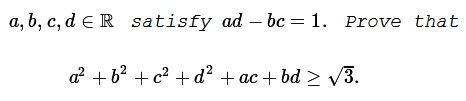# An Inequality in Parallelogram of Unit Area

### Problem### Solution 1

Define $A=\displaystyle\begin{pmatrix}a&c\\b&d\end{pmatrix}.\;$ Then $\det A=1,\;$ and so too $\det (A^tA)=1.\;$ But

$\displaystyle A^tA=\begin{pmatrix}a^2+b^2&ac+bd\\ac+bd&c^2+d^2\end{pmatrix},$

such that $(a^2+b^2)(c^2+d^2)=1+(ac+bd)^2,\;$ or $\sqrt{(a^2+b^2)(c^2+d^2)}=\sqrt{1+(ac+bd)^2}.\;$

Let $ac+bd=x.\;$ Then by the AM-GM inequality, $a^2+b^2+c^2+d^2\ge 2\sqrt{1+x^2}.\;$ Suffice it to show that $2\sqrt{1+x^2}+x\ge\sqrt{3}.$

If $x\gt\sqrt{3},\;$ there is nothing to prove. Else, if $x\le\sqrt{3},\;$ then the required inequality becomes have $(x\sqrt{3}+1)^2\ge 0,\;$ which is obviously true.

Note that the expression $|ad-bc|\;$ gives the area of the parallelogram build on vectors $\begin{pmatrix}a\\b\end{pmatrix}\;$ and $\begin{pmatrix}c\\d\end{pmatrix}.$

### Solution 2

Let $\overrightarrow{OA}=\left(\begin{array}\,a\\b\end{array}\right),\;$ $\overrightarrow{OB}=\left(\begin{array}\,c\\d\end{array}\right).\;$

Then, say,

\begin{align} T &= a^2+b^2+c^2+d^2+ac+bd\\ &= |\overrightarrow{OA}|^2+|\overrightarrow{OB}|^2+\overrightarrow{OA}\cdot \overrightarrow{OB}\\ &\ge 2|\overrightarrow{OA}||\overrightarrow{OB}|+\overrightarrow{OA}\cdot\overrightarrow{OB}, \end{align}

where the dot $\cdot\;$ denotes the scalar (dot) product. Let $U\;$ denotes the latter expression: $U=2|\overrightarrow{OA}||\overrightarrow{OB}|+\overrightarrow{OA}\cdot\overrightarrow{OB}.\;$ $T\ge U.$

On the other hand, $|ad-bc|=2[\Delta OAB],\;$ i.e., twice the area of $\Delta OAB,\;$ or the area of the parallelogram build on two vectors, $\overrightarrow{OA}\;$ and $\overrightarrow{OB}.\;$ It is easy to check that

$|ad-bc|^2=|\overrightarrow{OA}|^2|\overrightarrow{OB}|^2-\left(\overrightarrow{OA}\cdot\overrightarrow{OB}\right)^2,$

such that

$1+\left(\overrightarrow{OA}\cdot\overrightarrow{OB}\right)^2=|\overrightarrow{OA}|^2|\overrightarrow{OB}|^2,$

We can find angle $\theta\in\displaystyle\left(-\frac{\pi}{2},\frac{\pi}{2}\right)\;$ such that $\tan\theta=\overrightarrow{OA}\cdot\overrightarrow{OB}.\;$ Then $\displaystyle |\overrightarrow{OA}||\overrightarrow{OB}|=\frac{1}{\cos\theta},\;$ reducing $U\;$ to a function of $\theta:$

$\displaystyle U=U(\theta)=\frac{2}{\cos\theta}+\tan\theta.$

Since $\displaystyle U'(\theta)=\frac{2\sin\theta+1}{\cos^2\theta},\;$ the function has local minimum when $\sin\theta=-\frac{1}{2},\;$ i.e., when $\theta=\displaystyle -\frac{\pi}{6}.$

Kunihiko Chikaya has presented a beautiful geometric argument to support his answer. The expression $\displaystyle\frac{2}{\cos\theta}+\tan\theta,\;$ if written differently, clearly represents the slope of the line through $K=(0,-2)\;$ and $P(\cos\theta,\sin\theta),\;$ a point on the unit circle. Indeed, $\displaystyle\frac{2}{\cos\theta}+\tan\theta=\frac{\sin\theta-(-2)}{\cos\theta-0}.\;$ The slope of $KP\;$ is the smallest for $P=P'\;$ where $KP'\;$ is tangent to the circle:Obviously $\displaystyle P'=\left(\frac{\sqrt{3}}{2},-\frac{1}{2}\right)=\left(\cos (-\frac{\pi}{6}),\sin (-\frac{\pi}{6})\right).$

### Solution 3

As in Solution 2, we reduce the problem to another:

Find the minimum of $U=2|\overrightarrow{OA}||\overrightarrow{OB}|+\overrightarrow{OA}\cdot\overrightarrow{OB},\;$ subject to

$1+\left(\overrightarrow{OA}\cdot\overrightarrow{OB}\right)^2=|\overrightarrow{OA}|^2|\overrightarrow{OB}|^2,$

Introduce $x=|\overrightarrow{OA}||\overrightarrow{OB}|\;$ $y=\overrightarrow{OA}\cdot\overrightarrow{OB}.\;$ In terms of $x\;$ and $y\;$ the problem becomes

Find the minimum of $U(x,y)=2x+y,\;$ subject to $x\ge 0\;$ and

$1+y^2=x^2.$

This is equivalent to finding the tangent to the hyperbola $1=x^2-y^2\;$ of the form $2x+y=const.\;$ If $\min U=k,\;$ then, with $2x+y=k,\;$ we obtain a quadratic equation in $x:\;$ $3x^2-4kx+(k^2+1)=0.\;$For $k,\;$ this quadratic equation needs to have a unique solution, so that its discriminant ought to vanish: $(2k)^2-3(k^2+1)=0,\;$ wherefrom $k=\sqrt{3},\;$ since $k=-\sqrt{3}\;$ corresponds to the tangent to the other branch of the hyperbola where $x\lt 0.$

### Solution 4

Solution 3 could be branched into an application of the Lagrange multipliers by setting, say,

$U(x,y,\lambda) = 2x+y+\lambda(1+y^2-x^2).$

We have a system of equations with partial derivatives:

\begin{align} U_x &= 2-2\lambda x = 0\\ U_y &= 1 + 2\lambda y = 0\\ U_{\lambda} &= 1+y^2-x^2=0. \end{align}

Expressing $x\;$ and $y\;$ from the first two equations, $\displaystyle x=\frac{1}{\lambda}\;$ and $\displaystyle y=-\frac{1}{2\lambda},\;$ and substituting them into the third we find $\displaystyle\lambda^2=\frac{3}{4}.\;$ To have $x\;$ positive, we choose $\displaystyle\lambda=\frac{\sqrt{3}}{2}.\;$ For this $\lambda,\;$ $2x+y=\sqrt{3}.$

### Solution 5

Set $\overrightarrow{u}=\begin{pmatrix}a\\b\end{pmatrix}\;$ and $\overrightarrow{v}=\begin{pmatrix}c\\d\end{pmatrix},\;$ with $ad-bc=1.$

Rotating $\overrightarrow{u}\;$ amd $\overrightarrow{v}\;$ together does not change the value of

\begin{align}f(\overrightarrow{u},\overrightarrow{v})&=a^2+b^2+c^2+d^2+ac+bd\\ &=||\overrightarrow{u}||^2+||\overrightarrow{v}||^2+\overrightarrow{u}\cdot\overrightarrow{v}, \end{align}

nor the condition $ad-bc=1.\;$ Hence, WLOG, we can suppose that $\overrightarrow{u}\;$ is along the $x-\text{axis},\;$ that is $b=0.\;$ The expression of $f\;$ then takes a simple form:

$\displaystyle f(\overrightarrow{u},\overrightarrow{v})=a^2+c^2+\frac{1}{a^2}+ac=P_a(c).$

Since $P_a(X)=X^2+aX+a^2+\displaystyle\frac{1}{a^2}\;$ is a polynomial of degree 2 with positive leading coefficient, it admits a global minimum at $\displaystyle -\frac{a}{2},\;$ that is, for every $x\in\mathbb{R},$

$\displaystyle p_a(x)\ge P_a(-\frac{a}{2})=\frac{3a^2}{4}+\frac{1}{a^2}.$

We now apply the AM-GM inequality:

$\displaystyle \frac{3a^2}{4}+\frac{1}{a^2}\ge 2\sqrt{\frac{3}{4}}=\sqrt{3}.$

### Solution 6

By the AM-GM inequality, and t hen using the Lagrange identity

\begin{align} (a^2+b^2)+(c^2+d^2) &\ge 2\sqrt((a^2+b^2)(c^2+d^2))\\ &=2\sqrt{(ac+bd)^2+(ad-bc)^2}\\ &=2\sqrt{(ac+bd)^2+1}. \end{align}

It follows that

$a^2+b^2+c^2+d^2+ac+bd\ge 2\sqrt{(ac+bd)^2+1}+ac+bd.$

This, suffice it to prove that

$2\sqrt{(ac+bd)^2+1}+ac+bd\ge\sqrt{3}.$

But the latter is equivalent to

This, suffice it to prove that

(*)

$2\sqrt{(ac+bd)^2+1}\ge\sqrt{3}-{ac+bd}.$

Now, if $ac+bd\ge\sqrt{3},\;$ then (*) is obvious. So assume $ac+bd\lt\sqrt{3}.\;$ In this case, (*) is equivalent to

$\left[2\sqrt{(ac+bd)^2+1}\right]^2\ge\left[\sqrt{3}-(ac+bd)\right]^2.$

To continue,

$4\left[(ac+bd)^2+1\right]\ge\left[3+(ac+bd)^2-2\sqrt{3}(ac+bd)\right].$

And then

$3(ac+bd)^2+1-2\sqrt{3}(ac+bd)\ge 0,$

which is simply

$\left[\sqrt{3}(ac+bd)-1\right]^2\ge 0,$

and this is certainly true.

### Acknowledgment

Leo Giugiuc has kindly posted the problem and his solution (Solution 1) at the CuTheKnotMath facebook page, with a link to a mathematical inequalities facebook group. Following the link, I found that the problem has been posted much earlier, at which time Kunihiko Chikaya replied with a solution of his own, giving credit for the problem to Alim Eyvazov. I took the liberty to include his solution (Solution 2) in a slightly modified form. Kunihiko Chikaya then sent Solution 3 in a private message. Solution 5 has been posted on twitter.com by Lynx Myope; Solution 6 by Vaggelis Stamadiadis was pointed to by Kunihiko Chikaya.

### Linear Algebra Tools for Proving Inequalities

$\;\left(\displaystyle\left(\frac{a}{b-c}\right)^2+\left(\frac{b}{c-a}\right)^2+\left(\frac{c}{a-b}\right)^2\ge 2\right)$
• Linear Algebra Tools for Proving Inequalities: Cauchy-Binet Formula $\;\left(\displaystyle\left(\sum_{i=1}^{n}\frac{x_i^2}{a_i}\right)\cdot\left(\sum_{1\le i\lt j\le n}a_ia_j(x_iy_j-x_jy_i)^2\right)\ge \sum_{i=1}^{n}a_iy_i^2\right)$
• An Inequality from Gazeta Matematica, March 2016 (If $a^2+b^2+c^2=3\,$ then $(a+c)(1+b)\le 4)$
• An Inequality from Gazeta Matematica, March 2016 II (If $x^2+y^2+z^2+t^2=1\,$ then $\;(x+z)(y+t)\le 4)$
• An Inequality from Gazeta Matematica, March 2016 III $\;(a^2+b^2+1\ge a+ab+b)$
• An Inequality from Gazeta Matematica, March 2016 IV (If $a^2+b^2+c^2=1\,$ then $a+ac+b\le 2)$
• Problem 3980 from Crux Mathematicorum $\;\left(\displaystyle\sum_{cycl}\frac{a+b}{a-b}\prod_{cycl}\frac{a+b}{a-b}\lt\frac{1}{3}\right)$
• NonSquare Matrix as a Tool for Proving an Inequality $\;\left(2(a + b + c)((a + 2b + 3c) \ge (\sqrt{b(a+b)} + 2\sqrt{c(b+c)} + \sqrt{a(c+a)})^2\right)$
• An Inequality from a Vietnamese Problem Book $\;\left(\displaystyle \frac{a^3+2}{b+2c}+\frac{b^3+2}{c+2a}+\frac{c^3+2}{a+2b}\ge 3\right)$
• Hadamard's Determinant Inequalities and Applications I $\left((2-a-b-c+abc)^2\le (a^2+2)(b^2+2)(c^2+2)\right)$
• Hadamard's Determinant Inequalities and Applications II $\left((n + a - 1)(a - 1)^{n-1} \le a^n\right)$
•